# SAT Math Multiple Choice Question 948: Answer and Explanation

### Test Information

Question: 948

16. If u and v are complex numbers such that u = 3 – 5i and v = -6 + i, which of the following is equivalent to (u + v)2? (Note: i2 = -1)

• A.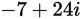• B.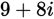• C.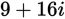• D.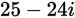Explanation:

A

Difficulty: Medium

Category: Additional Topics in Math / Imaginary Numbers

Strategic Advice: You could square (u + v) and then substitute the expressions given into the resulting product, but complex numbers add and subtract very nicely, so it is almost always quicker and easier to combine the complex numbers and then perform any additional operations.

Getting to the Answer: Start by substituting the expressions for u and v, combine them, and then square the result. You'll also need to use the fact that i2 = -1: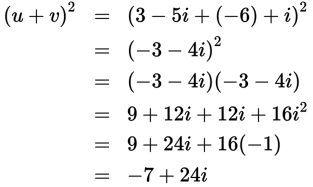This means (A) is correct.